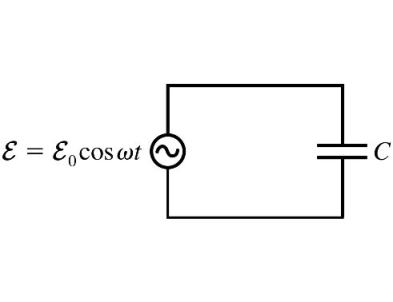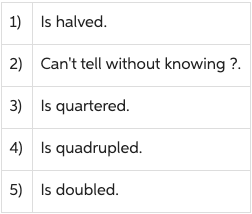# Problem: Part AIf the value of the capacitance is doubled, the capacitive reactance

###### FREE Expert Solution

Capacitive reactance:

$\overline{){{\mathbf{X}}}_{{\mathbf{C}}}{\mathbf{=}}\frac{\mathbf{1}}{\mathbf{\omega }\mathbf{C}}}$

98% (111 ratings)###### Problem Details

Part A

If the value of the capacitance is doubled, the capacitive reactance